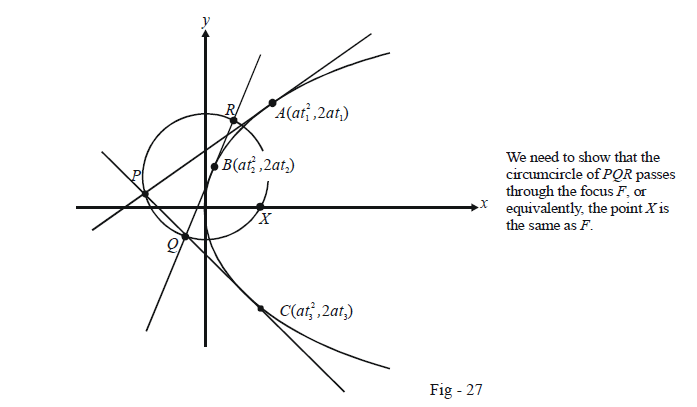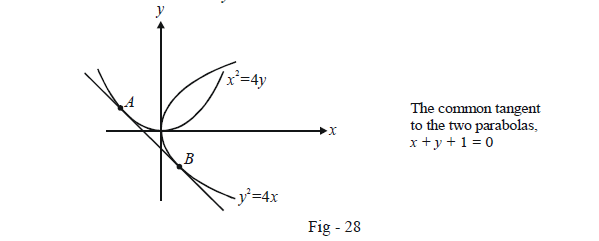# Examples on Tangents to Parabolas Set 2

Go back to  'Parabola'

Example – 20

Refer to Fig - 26 of Example 18 again. Show that angle FRP is a right angle.

Solution: Once again, we use the equation of the tangent at $$P(a{t^2},2at)$$ :

$ty = x + a{t^2}$

This intersects the y-axis at the point R(0, at). The slope of PR is simply the slope of the tangent at P, i.e. $${m_{PR}} = \frac{1}{t}$$

The slope of RF is

${m_{RF}} = \frac{{at - 0}}{{0 - a}} = - t$

Since $${m_{PR}} \times {m_{RF}} = - 1,$$ angle FRP is a right angle.

The result of the last three examples are important, so we summarize them here :

* (A) The tangent at any point on a parabola bisects the angle between the focal chord through that point and the perpendicular on the  directrix from that point (Example-18).

* (B) The portion of the tangent to a parabola cut-off between the directrix and the curve subtends a right angle at the focus (Example -19).

* (C) The perpendicular dropped from the focus onto any tangent to a parabola is concurrent with that tangent and the tangent at the vertex  (Example -20)

Along with these, we include a fourth important result here.

* (D) The tangents at the extremities of any focal chord of a parabola intersect at right angles on the directrix. (Example -16)

Example – 21

Three tangents to a parabola form the triangle PQR. Prove that the circumcircle of PQR passes through the focus of the parabola.

Solution: We assume the parabola to be $${y^2} = 4ax$$ and three points on it to be $$A(at_1^2,2a{t_1}),\,B(at_2^2,2a{t_2})$$ and $$C(at_3^2,2a{t_3}).$$ Tangents at A, C and B meet at P, Q and R as shown:The co-ordinates of the points P, Q and R are respectively $(a{t_1}{t_3},\,a({t_1} + {t_2})),\,\,(a{t_2}{t_3},\,a({t_2} + {t_3})\;\rm{}and\;(a{t_1}{t_2},\,a({t_1} + {t_2})).$

To show that the circumcircle passes through F, it would suffice to prove that the chord PQ subtends the same angle on F as it does on R. Since a chord of a circle subtends equal angles anywhere on the circumference, this will prove that F also lies on the circumference of the circle.

To evaluate $$\angle PRQ,$$ we need the slopes of PR and RQ which are simply the slopes of the tangents at A and B respectively, i.e.

${m_{PR}} = \frac{1}{{{t_1}}}{\rm{ }} \;and\;\;{\rm{ }}{m_{RQ}} = \frac{1}{{{t_2}}}$

Thus,

\begin{align}\tan (\angle PRQ)&= \frac{{{m_{PR}} - {m_{RQ}}}}{{1 + {m_{PR}}{m_{RQ}}}}\\\\&= \frac{{{t_2} - {t_1}}}{{1 + {t_1}{t_2}}} \qquad \qquad \dots \left( 1 \right)\end{align}

Now, to evaluate the angle that the chord PQ subtends at F, we need to slopes $${m_{PF}}{\rm{ }}\;{\rm{}and}\;{\rm{ }}{m_{QF}}$$:

\begin{align}&\qquad \quad \;\;{m_{PF}} = \frac{{a({t_1} + {t_3})}}{{a{t_1}{t_3} - a}}\;{\rm{ }}and\;{\rm{ }}{m_{QF}} = \frac{{a({t_2} + {t_3})}}{{a{t_2}{t_3} - a}}\\\\&\Rightarrow \qquad {m_{PF}} = \frac{{{t_1} + {t_3}}}{{{t_1}{t_3} - 1}}{\text{ }}\;and\;{\text{ }}{m_{QF}} = \frac{{{t_2} + {t_3}}}{{{t_2}{t_3} - 1}}\end{align}

Thus,

\begin{align}& \tan (\angle PFQ) = \frac{{{m_{PF}} - {m_{QF}}}}{{1 + {m_{PF}}{m_{QF}}}}\\\\&\qquad\qquad\quad\;= \frac{{({t_1} + {t_3})({t_2}{t_3} - 1) - ({t_2} + {t_3})({t_1}{t_3} - 1)}}{{({t_1}{t_3} - 1)({t_2}{t_3} - 1) + ({t_1} + {t_3})({t_2} + {t_3})}}\\\\&\qquad\qquad\quad\;= \frac{{{t_2} - {t_1}}}{{1 + {t_1}{t_2}}} \qquad \qquad \qquad \qquad\qquad\qquad\qquad \dots \left( 2 \right)\end{align}

Comparing (1) and (2) gives $$\angle PRQ = \angle PFQ$$ which confirms that F does indeed lie on the circumference of $$\Delta PQR's$$ circumcircle.

We could alternatively have done this question by explicitly evaluating the equation of the circle passing through P, Q and R and showing the F satisfies that equation.

Example - 22

Find the equation of the common tangent(s) to

(a) $${y^2} = 4ax\;\;\text{and}\;\;{(x + a)^2} + {y^2} = {a^2}$$

(b) $${y^2} = 4x\;\text{and}\;\;{x^2} = 4y$$

Solution: (a) The equation of any tangent to $${y^2} = 4ax$$ can be written as

$$ty = x + a{t^2}$$

If this is to touch the circle too, the distance of the circle’s centre, i.e. (a, 0) from this line must be equal to its radius a. Thus,

\begin{align}& \qquad \frac{{\left| { - a + a{t^2}} \right|}}{{\sqrt {1 + {t^2}} }} = a\\\\& \qquad {(1 - {t^2})^2} = (1 + {t^2})\\\\&\Rightarrow \qquad 1 + {t^4} - 2{t^2} = 1 + {t^2}\\\\&\Rightarrow \qquad {t^2}({t^2} - 3) = 0\\\\&\Rightarrow \qquad t = \pm \sqrt 3 \left( {t = {\rm{ 0\;is\;not\;a\;feasible\;solution\;for\;this\;case}}} \right)\end{align}

Thus, the required common tangents are

$\pm \sqrt 3 y = x + 3a$

(b) Any tangent to $${y^2} = 4x$$ can be assumed to be of the form

$ty = x + {t^2}\left( {{\rm{since\; }}{\rm{ }}a = {\rm{ }}1{\rm{\;for\;this\;case}}} \right)$

If this line is to be tangent to $${x^2} = 4y$$ too, its intersection with this curve must yield only one point. Thus, $t\left( {\frac{{{x^2}}}{4}} \right) = x + {t^2}$ must yield only one root, i.e. its D must equal O.

This gives

\begin{align}& \qquad \;\;16 + 16{t^3} = 0\\&\Rightarrow \quad t = - 1\end{align}

The required common tangent is thus

$x + y + 1 = 0$